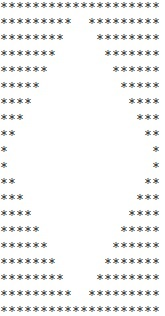# C program to print diamond pattern

In this C program, we are going to learn how to create a diamond pattern of asterisks? Here, we are creating a diamond pattern for that we are using 10 fixed rows.
Submitted by Ashish Varshney, on March 02, 2018

Following image is showing a diamond pattern,Here, we are printing a diamond using c program, for this we are taking 10 numbers of rows and using one parent loop to print its upper half diamond pattern and three child loops 1) to print asterisks before space, 2) to print space and 3) to print asterisks apace space.

Same as, there is another parent loop with same as above child loops to print its lower half diamond.

## Program to print diamond in C language

﻿

```#include <sdtio.h>

int main()
{
int row,column,Space;
//Loop to print first-half pattern
for(row=0;row<10;row++)
{
for(column=0;column<10-row;column++)
printf("*");

for(Space=0;Space<2*row;Space++)
printf(" ");

for(column=0;column<10-row;column++)
printf("*");

if(row<9)
printf("\n");
}

//Loop to print second-half pattern
for(row=0;row<=10;row++)
{
for(column=0;column<10-row;column++)
printf("*");

for(Space=0;Space<2*(10-row);Space++)
printf(" ");

for(column=0;column<10-row;column++)
printf("*");

printf("\n");
}

return 0;
}
```